# NCERT Solutions, Matrices, Part -4, Class 12, Maths Notes - Class 12

## Class 12: NCERT Solutions, Matrices, Part -4, Class 12, Maths Notes - Class 12

The document NCERT Solutions, Matrices, Part -4, Class 12, Maths Notes - Class 12 is a part of Class 12 category.
All you need of Class 12 at this link: Class 12

Matrices

Exercise 3.4
Question 1:
Find the inverse of each of the matrices, if it exists.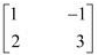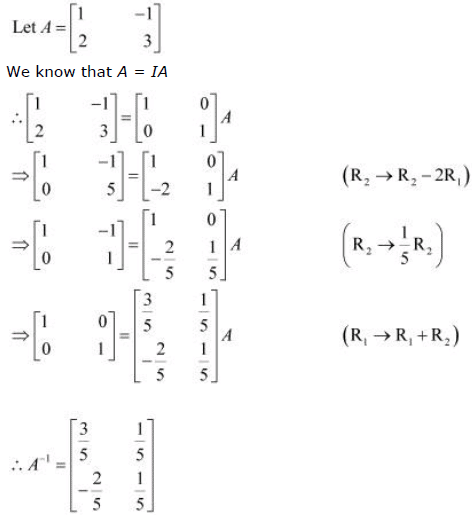Question 2: Find the inverse of each of the matrices, if it exists.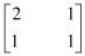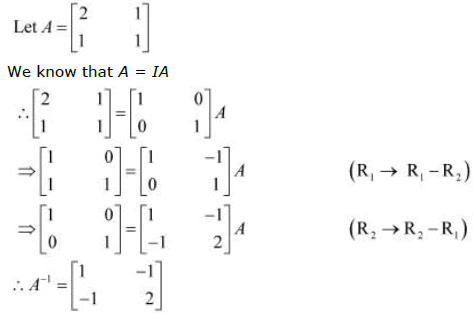Question 3: Find the inverse of each of the matrices, if it exists.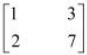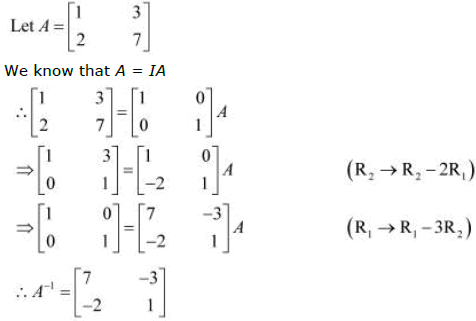Question 4: Find the inverse of each of the matrices, if it exists.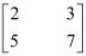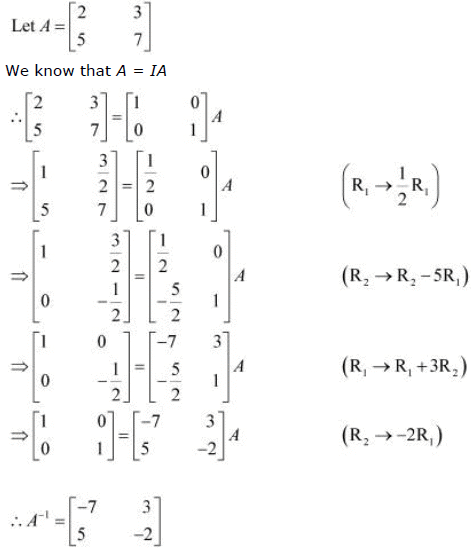Question 5: Find the inverse of each of the matrices, if it exists.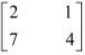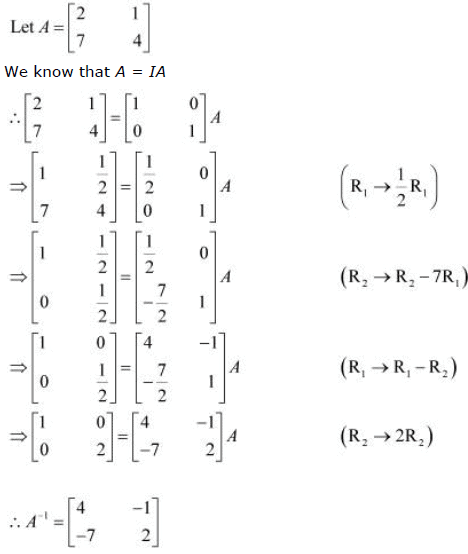Question 6: Find the inverse of each of the matrices, if it exists.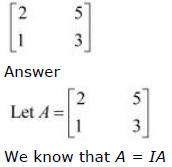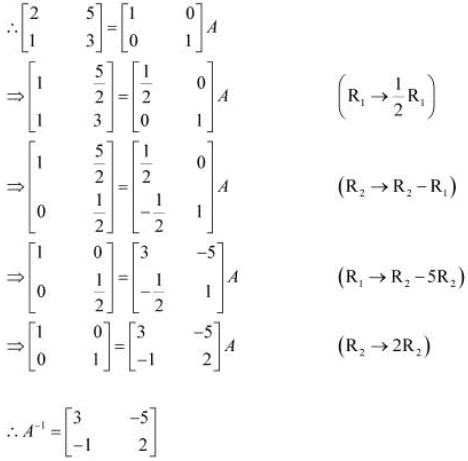Question 7: Find the inverse of each of the matrices, if it exists.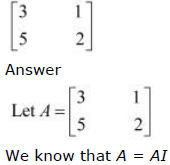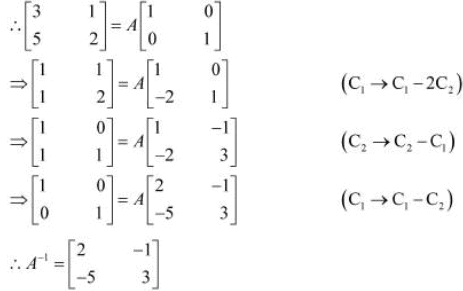Question 8: Find the inverse of each of the matrices, if it exists.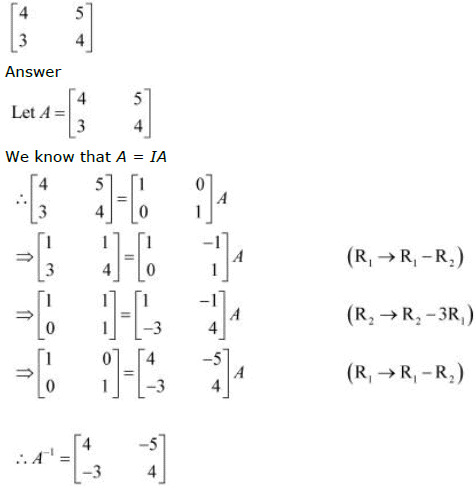Question 9: Find the inverse of each of the matrices, if it exists.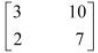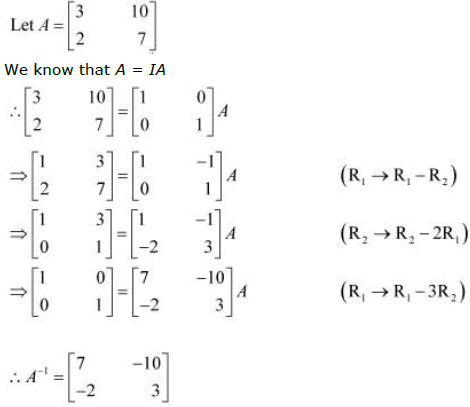Question 10: Find the inverse of each of the matrices, if it exists.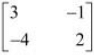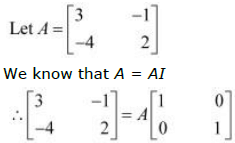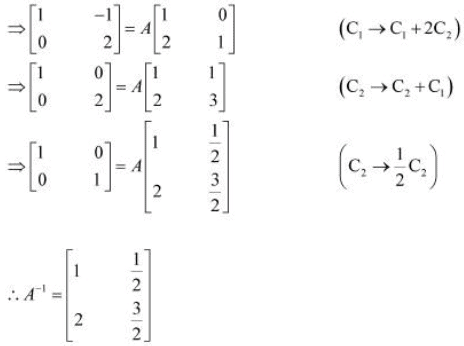Question 11: Find the inverse of each of the matrices, if it exists.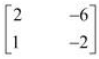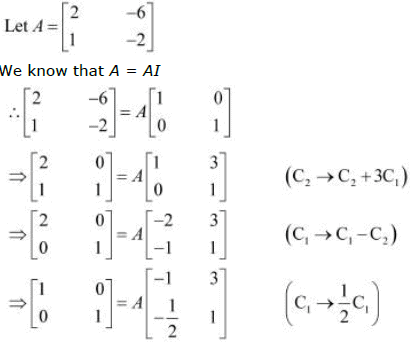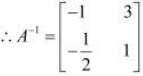Question 12: Find the inverse of each of the matrices, if it exists.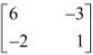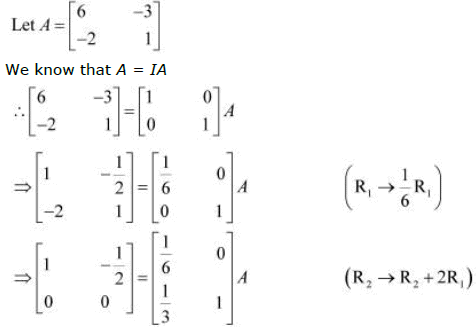Now, in the above equation, we can see all the zeros in the second row of the matrix on
the L.H.S.
Therefore, A−1 does not exist.

Question 13: Find the inverse of each of the matrices, if it exists.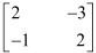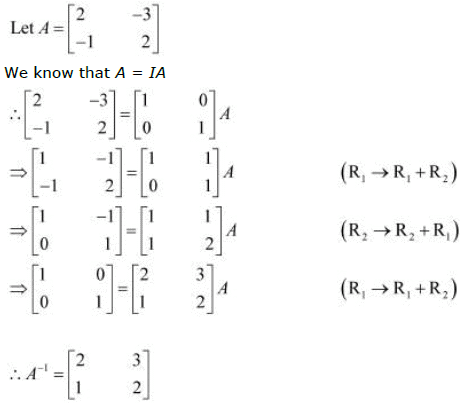Question 14: Find the inverse of each of the matrices, if it exists.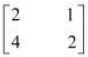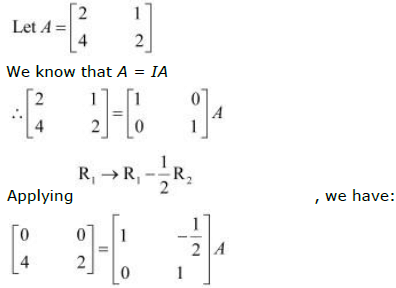Now, in the above equation, we can see all the zeros in the first row of the matrix on the
L.H.S.
Therefore, A−1 does not exist.

Question 16: Find the inverse of each of the matrices, if it exists.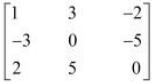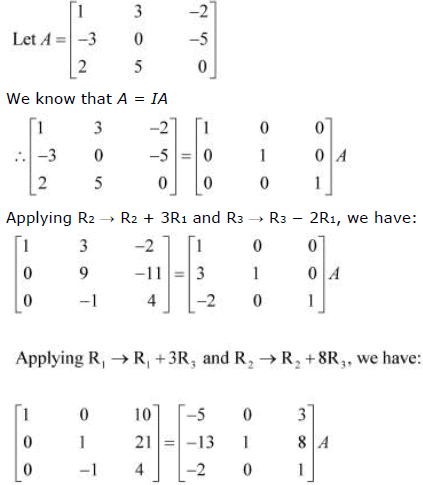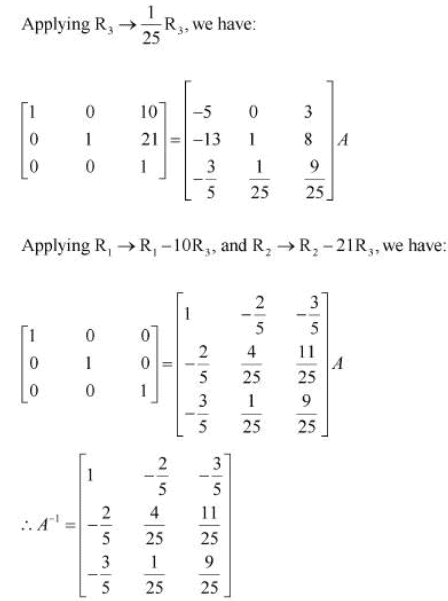Question 17: Find the inverse of each of the matrices, if it exists.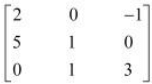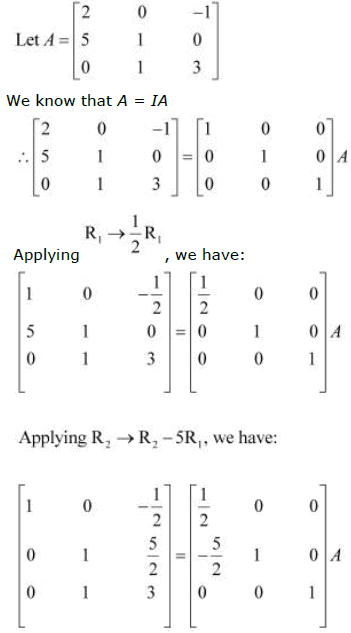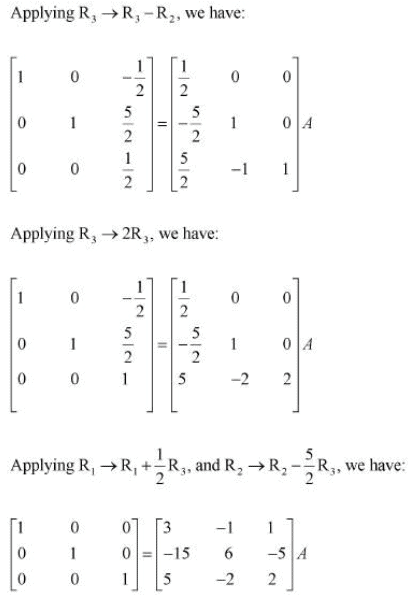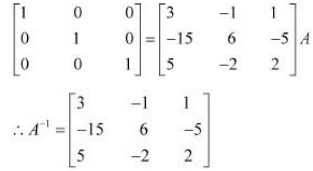Question 18: Matrices A and B will be inverse of each other only if
A. AB = BA

B. AB = BA = 0
C. AB = 0, BA = I

D. AB = BA = I

We know that if A is a square matrix of order m, and if there exists another square matrix
B of the same order m, such that AB = BA = I, then B is said to be the inverse of A. In
this case, it is clear that A is the inverse of B.
Thus, matrices A and B will be inverses of each other only if AB = BA = I.

The document NCERT Solutions, Matrices, Part -4, Class 12, Maths Notes - Class 12 is a part of Class 12 category.
All you need of Class 12 at this link: Class 12Use Code STAYHOME200 and get INR 200 additional OFF

Track your progress, build streaks, highlight & save important lessons and more!

,

,

,

,

,

,

,

,

,

,

,

,

,

,

,

,

,

,

,

,

,

,

,

,

,

,

,

,

,

,

,

,

,

;